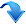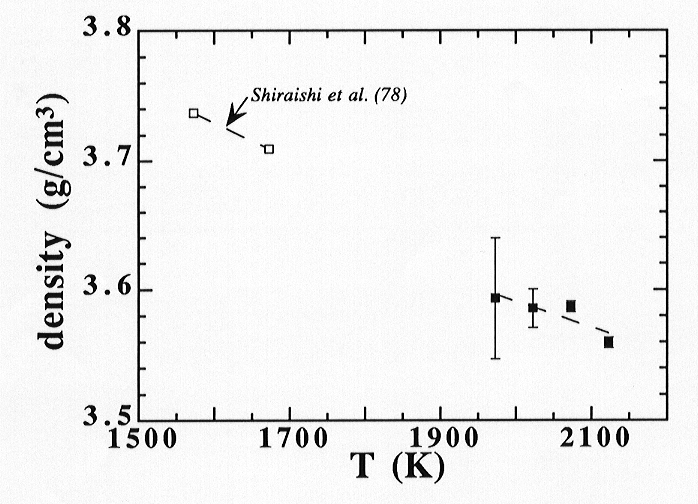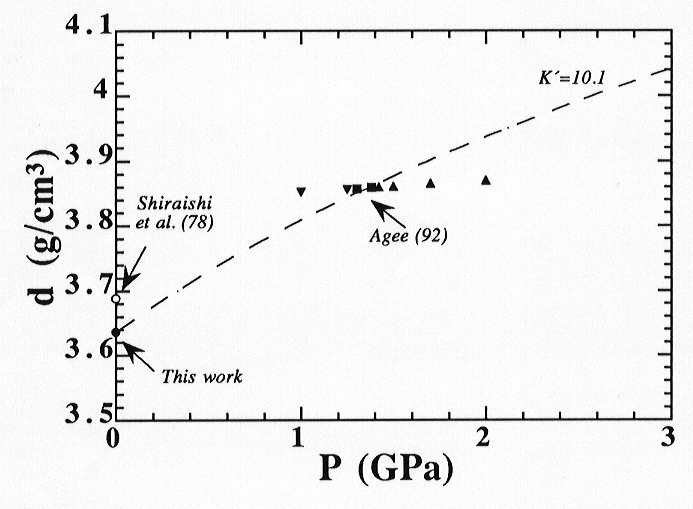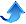3.6 h. High-temperature density of fayalite melt (P. Courtial and D.B. Dingwell)

Understanding the evolution of the Earth´s interior via magmatic processes requires precise high-pressure high-temperature data on densities of silicate melts, especially of fayalite melt since fayalite melt represents a major constituent of most natural magmas. Combined with high-pressure densities from static determinations, experimental one-atmosphere densities provide a firm constraint for extrapolation of the densities of magmatic melts to higher pressures.

One-atmosphere density measurements in equilibrium with air and at high temperatures were thus conducted on fayalite melt using the Ir-based double-bob Archimedean method. Densities of the present mantle melt range from 3.59 to 3.56 g/cm3 over temperatures ranging from 1973 to 2123 K as plotted in Fig. 3.6-8. Experimental densities are quite well documented for this compound, but most of the previous studies were conducted using the "maximum bubble pressure" method. In fact, it has been proposed that discrepancies can be caused by the oxygen pressure in the bubble forming gas and the temperature inhomogeneity of the melt in such a "maximum bubble pressure" method. On the other hand, Shiraishi et al., 1978 (Trans. Jap. Inst. Metal., 19, 264) have reported their density data performed in an argon atmosphere using the double-bob Archimedean method over a temperature interval ranging from 1573 to 1673 K, also plotted in Fig. 3.6-8, in which bobs and crucibles were iron. Their measurements were performed at temperatures much lower than ours because these two studies were made at different redox conditions (i.e., under argon for Shiraishi et al. and under air in this work). Shiraishi et al. estimated their sample to be almost fully reduced, whereas the ferric/ferrous ratio calculated from literature models suggests 0.46 at 1973 K and 0.33 at 2073 K. Extrapolation of the data of Shiraishi et al. to the temperature range investigated in this study match the present one well, even though these two studies suggest slightly different thermal expansion coefficients. Based on two experiments at temperatures differing more than 100 K, Shiraishi et al. calculated a thermal expansion coefficient for fayalite melt of 7.13 x 10-5 K-1, which is larger than the one obtained in the present study (i.e., 5.7 x 10-5 K-1), assuming that all the Fe is as FeO within the temperature range investigated.Fig. 3.6-8: One-atmosphere experimental densities of molten fayalite. In addition, the densities measurements of Shiraishi et al., 1978 are also plotted.

Together with the high-pressure measurements of Agee 1992 (G.R.L., 19, 1169), the present one-atmosphere experiments allow straightforward interpolations in a large area of P-T space and thus provide firm constraints on the isothermal compression curves. Fitting the third order Birch-Murnaghan equation of state to both the high-pressure densities and the new one-atmosphere densities yield a new isothermal compression curve for molten fayalite (Fig. 3.6-9), in which the unknown parameters are the isothermal bulk modulus (KT) and its pressure derivative (T). A new value for the isothermal bulk modulus of 17.2 GPa is found at 1773 K taking the same pressure derivative of 10.1 as Agee. Agee determined previously an isothermal bulk modulus of 24.4 GPa and its pressure derivative of 10.1 for fayalite melt at 1773 K by constraining its high-pressure data to one atmosphere with the density measurements of Shiraishi et al. This present KT is 30% smaller than the one obtained by Agee.Fig. 3.6-9: The isothermal compression curve at 1773 K of molten fayalite calculated from the third order Birch-Murnaghan equation of state using static experiments of Agee, 1992. The static results have been reported by an arrow up, an arrow down and a square indicating the floating, the sinking and the neutral buoyancy of density markers, respectively. One-atmosphere experimental density of Shiraishi et al., 1978 extrapolated to 1773 K is also plotted.Bayerisches Geoinstitut, University of Bayreuth, 95440 Bayreuth, Germany
Tel: +49-(0) 921 55 3700 / 3766, Fax: +49-(0) 921 55 3769, E-mail: bayerisches.geoinstitut(at)uni-bayreuth.de